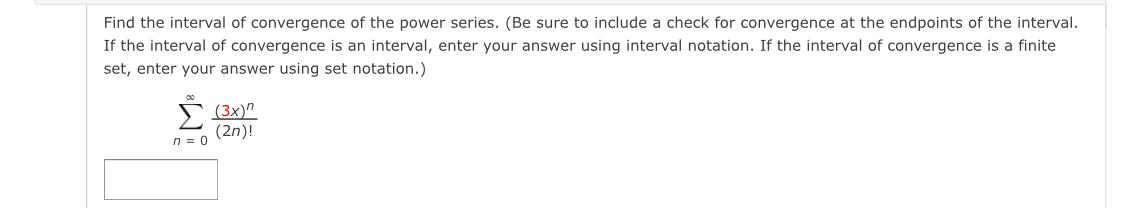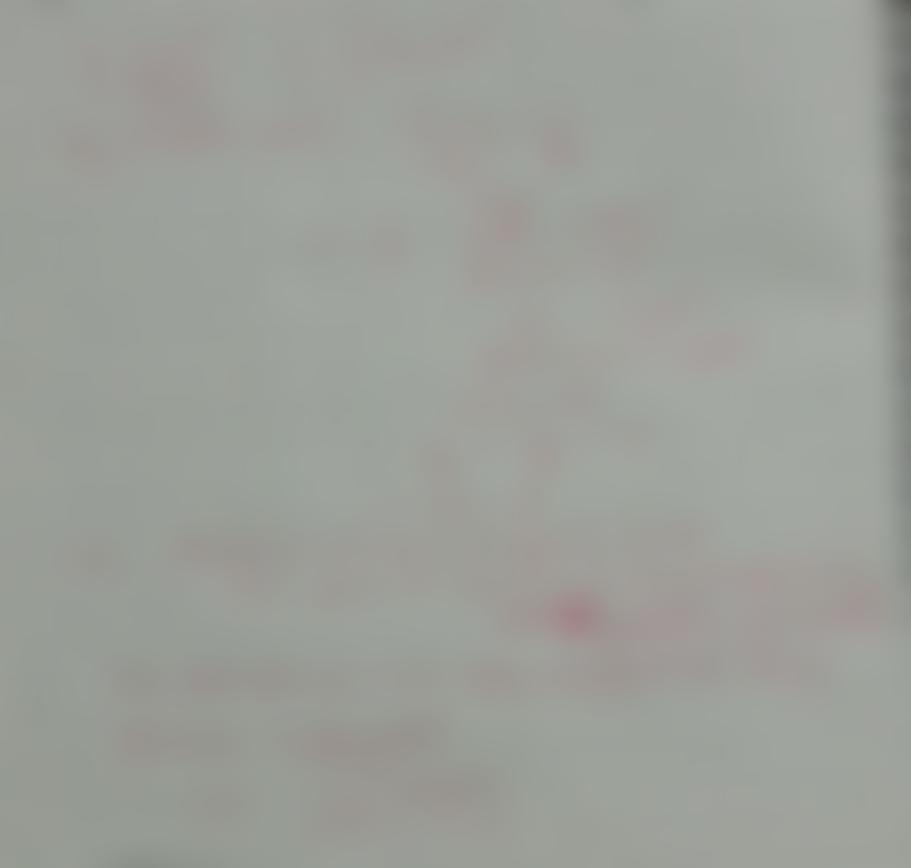Question:

Find the interval of convergence of the power series. (Be sure to include a check for convergence at the endpoints of the intervFind the interval of convergence of the power series. (Be sure to include a check for convergence at the endpoints of the interval. If the interval of convergence is an interval, enter your answer using interval notation. If the interval of convergence is a finite set, enter your answer using set notation.) § (3x)" (2n)! n = 0